## A Christmas Theorem

at 8:01 p.m. on 22. December, 2013

Theorem. Santa Claus exists.

Proof. Let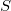be the sentence "Ifthen Santa Claus exists." This looks a bit self-referential but actually such a sentence, or an equivalent one, is constructible by the Diagonalisation Lemma.

Now,is of the form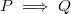where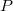(the antecedent) is justand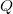(the consequent) is "Santa Claus exists." So of course if we assumeand show thatis true, then we have showed. Hence assume. Hence (by) Santa Claus exists. But this was the consequent. Hence. Hence Santa Claus exists.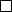Corollary. Merry Christmas!

Related tags: christmas humour maths theorem